# Introduction

First, let's create explainers for random Forest model and linear model

``````library("DALEX")
library("randomForest")
library("ceterisParibus")
set.seed(59)

# set default theme
theme_set(theme_bw() + theme(text = element_text(size = 18)))

# preprare model
apartments_rf_model <- randomForest(m2.price ~ construction.year + surface + floor +
no.rooms + district,
data = apartments)

explainer_rf <- explain(apartments_rf_model,
data = apartmentsTest[,2:6],
y = apartmentsTest\$m2.price)
``````

# Simple plots

``````apartments_A <- apartmentsTest[958,]
cp_rf_A <- ceteris_paribus(explainer_rf, apartments_A, y = apartments_A\$m2.price)

plot(cp_rf_A, show_profiles = TRUE, show_observations = TRUE,
selected_variables = c("surface","construction.year"))
``````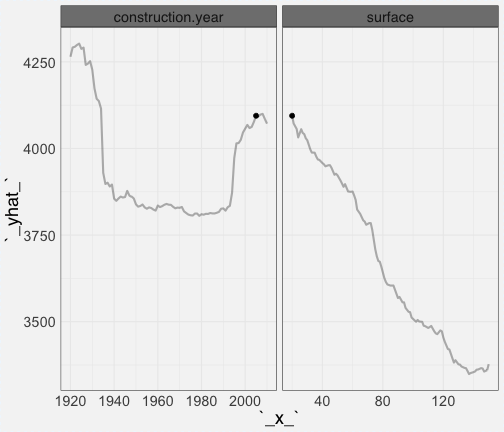Here are plots for neighbours of this observation.

``````apartments_B <- select_neighbours(apartmentsTest, apartmentsTest[958,], n = 15)
cp_rf_B <- ceteris_paribus(explainer_rf, apartments_B, y = apartments_B\$m2.price)

plot(cp_rf_B,
show_profiles = TRUE, show_observations = TRUE, show_rugs = TRUE,
selected_variables = c("surface","construction.year"))
``````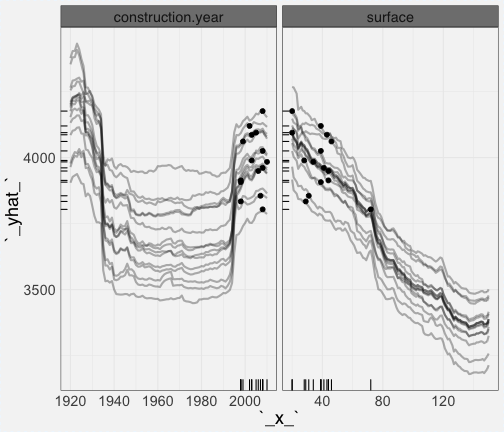Here we have an example for a subset of observations and only points.

``````apartments_C <- select_sample(apartmentsTest, n = 15)
cp_rf_C <- ceteris_paribus(explainer_rf, apartments_C, y = apartments_C\$m2.price)

plot(cp_rf_C,
show_profiles = FALSE,
selected_variables = c("surface","construction.year"))
``````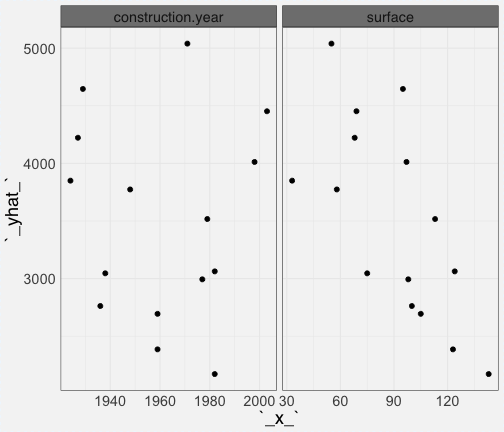Only rugs

``````plot(cp_rf_C,
show_profiles = FALSE, show_observations = FALSE, show_rugs = TRUE,
selected_variables = c("surface","construction.year"))
``````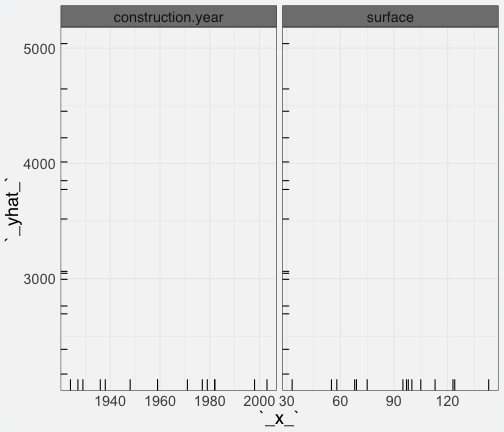Only profiles

``````plot(cp_rf_C,
show_profiles = TRUE, show_observations = FALSE,
selected_variables = c("surface","construction.year"))
``````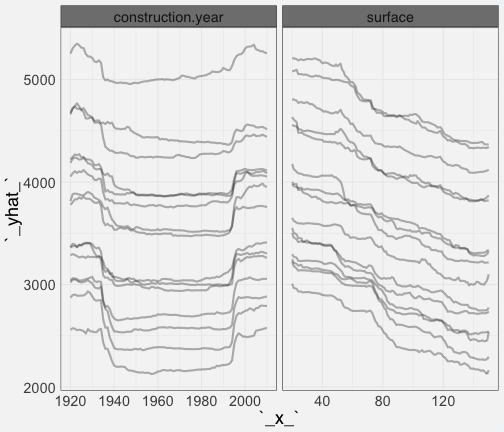Only residuals

``````plot(cp_rf_C,
show_profiles = FALSE, show_observations = FALSE, show_residuals = TRUE,
selected_variables = c("surface","construction.year"))
``````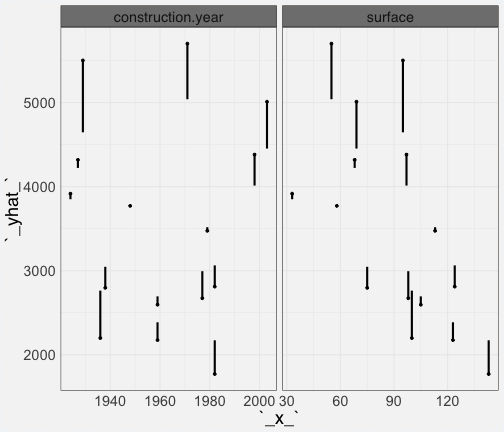Only average profile

``````plot(cp_rf_C,
show_profiles = TRUE, show_observations = FALSE,
aggregate_profiles = mean, size = 2,
selected_variables = c("surface","construction.year"))
``````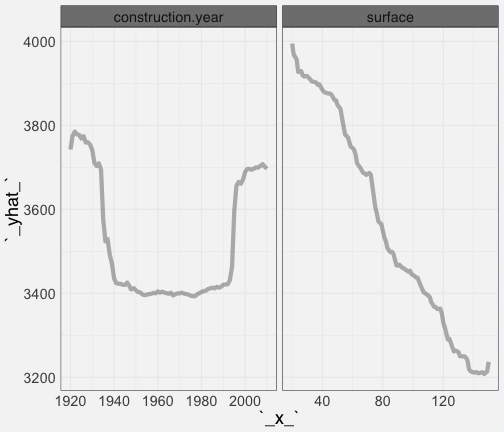Colors correspond to district

``````plot(cp_rf_C,
show_profiles = TRUE, show_observations = FALSE,
color = "district", alpha = 1,
selected_variables = c("surface","construction.year", "district"))
``````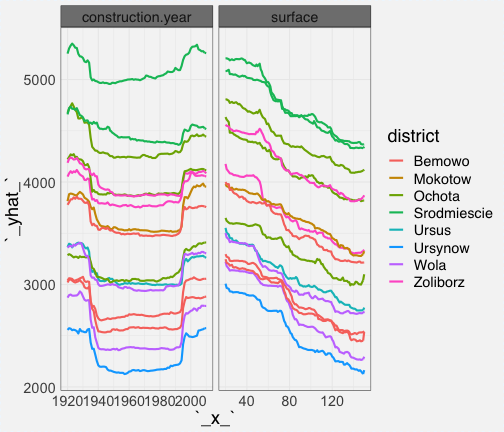Colors correspond to surface.

``````plot(cp_rf_C,
show_profiles = TRUE, show_observations = TRUE,
color = "surface", alpha = 1,
selected_variables = c("surface","construction.year"))
``````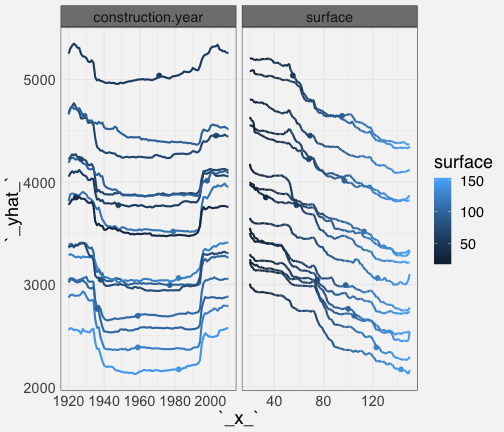Observations without rugs.

``````plot(cp_rf_C,
show_profiles = TRUE, show_observations = TRUE,
selected_variables = c("surface","construction.year"))
``````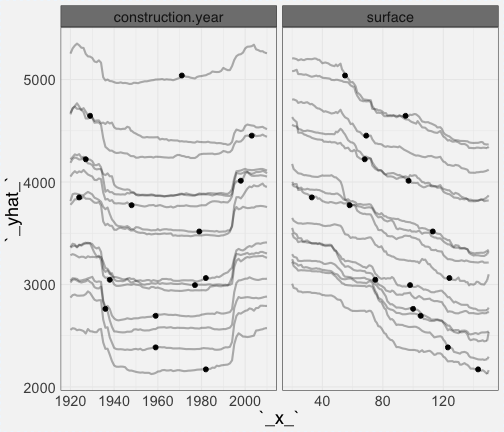Different colors for different elements.

``````plot(cp_rf_C,
show_profiles = TRUE, show_observations = TRUE, show_rugs = TRUE,
show_residuals = TRUE,
color = "blue", color_points = "orange", color_residuals = "red", color_rugs = "green",
alpha = 0.3, alpha_points = 0.3, alpha_residuals = 0.5, alpha_rugs = 1,
size_points = 4, size_rugs = 0.5,
selected_variables = c("surface","construction.year"))
``````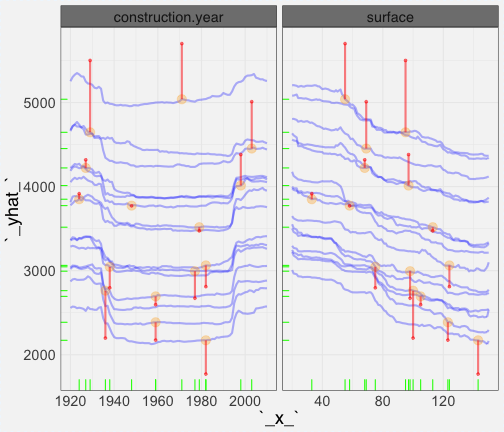# Complex plots

Here we are going to mix two or more layers.

First one presents individual profiles and the average between profiles.

``````# mixtures

plot(cp_rf_C,
show_observations = FALSE, show_rugs = TRUE,
show_residuals = TRUE, color_residuals = "red", size_residuals = 2,
selected_variables = c("surface","construction.year")) +
ceteris_paribus_layer(cp_rf_C,
show_observations = FALSE, show_rugs = FALSE,
aggregate_profiles = mean, size = 2, alpha = 1,
selected_variables = c("surface","construction.year"))
``````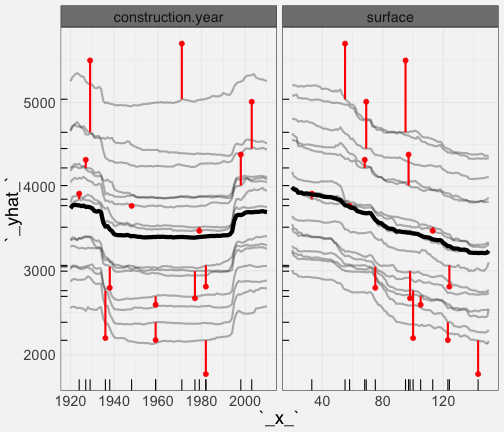Neighbourings for two observations.

``````apartments_D <- select_neighbours(apartmentsTest, apartmentsTest[348,], n = 15)
cp_rf_D <- ceteris_paribus(explainer_rf, apartments_D, y = apartments_D\$m2.price)

plot(cp_rf_B,
show_observations = FALSE, show_residuals = TRUE,
color_residuals = "blue",
selected_variables = c("surface","construction.year")) +
ceteris_paribus_layer(cp_rf_D,
show_observations = FALSE, show_residuals = TRUE,
color = "orange", color_residuals = "red",
selected_variables = c("surface","construction.year"))
``````